# ${\left(\phi ,\alpha ,\delta ,\lambda ,\mathrm{\Omega }\right)}_{p}$-Neighborhood for some classes of multivalent functions

## Abstract

In the present paper, we obtain some interesting results for neighborhoods of multivalent functions. Furthermore, we give an application of Miller and Mocanu’s lemma.

MSC:30C45.

## 1 Introduction and definitions

Let A denote the class of functions f of the form

$f\left(z\right)=z+\sum _{n=2}^{\mathrm{\infty }}{a}_{n}{z}^{n}$

which are analytic in the open unit disk

We denote by $A\left(p,n\right)$ the class of functions f of the form

$f\left(z\right)={z}^{p}+\sum _{k=n}^{\mathrm{\infty }}{a}_{k+p}{z}^{k+p}\phantom{\rule{1em}{0ex}}\left(n,p\in \mathbb{N}=\left\{1,2,\dots \right\}\right)$

which are analytic and multivalent in the open unit disk U.

The concept of neighborhood for $f\in A$ was first given by Goodman . The concept of δ-neighborhoods ${N}_{\delta }\left(f\right)$ of analytic functions $f\in A$ was first introduced by Ruscheweyh . Walker  defined a neighborhood of analytic functions having positive real part. Owa et al.  generalized of the results given by Walker. In 1996, Altıntaş and Owa  gave $\left(n,\delta \right)$-neighborhoods for functions $f\in A$ with negative coefficients. In 2007, new definitions for neighborhoods of analytic functions $f\in A$ were considered by Orhan et al. . The authors gave the following definition of neighborhoods:

For $f,g\in A$, f is said to be $\left(\alpha ,\delta \right)$-neighborhood for g if it satisfies

$|{f}^{\prime }\left(z\right)-{e}^{i\alpha }{g}^{\prime }\left(z\right)|<\delta \phantom{\rule{1em}{0ex}}\left(z\in U\right)$

for some $-\pi \le \alpha \le \pi$ and $\delta >\sqrt{2\left(1-cos\alpha \right)}$. They denote this neighborhood by $\left(\alpha ,\delta \right)-N\left(g\right)$.

Also, they saw that $f\in \left(\alpha ,\delta \right)-M\left(g\right)$ if it satisfies

$|\frac{f\left(z\right)}{z}-{e}^{i\alpha }\frac{g\left(z\right)}{z}|<\delta \phantom{\rule{1em}{0ex}}\left(z\in U\right)$

for some $-\pi \le \alpha \le \pi$ and $\delta >\sqrt{2\left(1-cos\alpha \right)}$.

In 2009, Altuntaş et al.  gave the following definition for neighborhood of analytic functions $f\in A\left(p,n\right)$.

For $f,g\in A\left(p,n\right)$, f is said to be ${\left(\alpha ,\delta \right)}_{p}$-neighborhood for g if it satisfies

$|\frac{{f}^{\prime }\left(z\right)}{{z}^{p-1}}-{e}^{i\alpha }\frac{{g}^{\prime }\left(z\right)}{{z}^{p-1}}|<\delta \phantom{\rule{1em}{0ex}}\left(z\in U\right)$

for some $-\pi \le \alpha \le \pi$ and $\delta >p\sqrt{2\left(1-cos\alpha \right)}$. They denote this neighborhood by ${\left(\alpha ,\delta \right)}_{p}-N\left(g\right)$.

Also, they saw that $f\in {\left(\alpha ,\delta \right)}_{p}-M\left(g\right)$ if it satisfies

$|\frac{f\left(z\right)}{{z}^{p}}-{e}^{i\alpha }\frac{g\left(z\right)}{{z}^{p}}|<\delta \phantom{\rule{1em}{0ex}}\left(z\in U\right)$

for some $-\pi \le \alpha \le \pi$ and $\delta >\sqrt{2\left(1-cos\alpha \right)}$.

Recently, Frasin  introduced the following definition of $\left(\alpha ,\beta ,\delta \right)$-neighborhood for analytic function f in the form

$f\left(z\right)=z-\sum _{n=2}^{\mathrm{\infty }}{a}_{n}{z}^{n}\phantom{\rule{1em}{0ex}}\left({a}_{n}\ge 0\right).$
(1.1)

Let f be defined by (1.1). Then f is said to be $\left(\alpha ,\beta ,\delta \right)$-neighborhood for $g=z-{\sum }_{n=2}^{\mathrm{\infty }}{b}_{n}{z}^{n}$ (${b}_{n}\ge 0$) if it satisfies

$|{e}^{i\alpha }{\left({D}^{k}f\left(z\right)\right)}^{\prime }-{e}^{i\beta }{\left({D}^{k}g\left(z\right)\right)}^{\prime }|<\delta$

for some $-\pi \le \alpha ,\beta \le \pi$ and $\delta >\sqrt{2\left(1-cos\left(\alpha -\beta \right)\right)}$.

The differential operator ${D}^{k}$ was introduced by Salagean .

Now, we give the following equalities for the functions $f\in A\left(p,n\right)$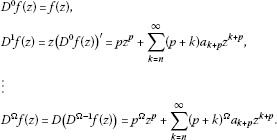We define $\mathrm{\wp }:A\left(p,n\right)\to A\left(p,n\right)$ such that

$\mathrm{\wp }\left(f\left(z\right)\right)=\left(\frac{1}{{p}^{\mathrm{\Omega }}}-\lambda \right){D}^{\mathrm{\Omega }}f\left(z\right)+\frac{\lambda }{p}z{\left({D}^{\mathrm{\Omega }}f\left(z\right)\right)}^{\prime }\phantom{\rule{1em}{0ex}}\left(0\le \lambda \le \frac{1}{{p}^{\mathrm{\Omega }}},\mathrm{\Omega }\in \mathbb{N}\cup \left\{0\right\}\right).$
(1.2)

We denote by ${\mathrm{\wp }}_{\left(\mathrm{\Omega },\lambda \right)}$ the class of analytic functions of the form (1.2) in U.

For $f,g\in {\mathrm{\wp }}_{\left(\mathrm{\Omega },\lambda \right)}$, f is said to be ${\left(\phi ,\alpha ,\delta ,\lambda ,\mathrm{\Omega }\right)}_{p}$-neighborhood for g if it satisfies

$|{e}^{i\phi }\frac{{\mathrm{\wp }}^{\mathrm{\prime }}\left(f\left(z\right)\right)}{{z}^{p-1}}-{e}^{i\alpha }\frac{{\mathrm{\wp }}^{\mathrm{\prime }}\left(g\left(z\right)\right)}{{z}^{p-1}}|<\delta \phantom{\rule{1em}{0ex}}\left(z\in U\right)$

for some $-\pi \le \phi -\alpha \le \pi$ and $\delta >p\sqrt{2\left(1-cos\left(\phi -\alpha \right)\right)}$. We denote this neighborhood by ${\left(\phi ,\alpha ,\delta ,\lambda ,\mathrm{\Omega }\right)}_{p}-\mathcal{N}\left(g\right)$.

Also, we say that $f\in {\left(\phi ,\alpha ,\delta ,\lambda ,\mathrm{\Omega }\right)}_{p}-\mathcal{M}\left(g\right)$ if it satisfies

$|{e}^{i\phi }\frac{\mathrm{\wp }\left(f\left(z\right)\right)}{{z}^{p}}-{e}^{i\alpha }\frac{\mathrm{\wp }\left(g\left(z\right)\right)}{{z}^{p}}|<\phantom{\rule{0.25em}{0ex}}\delta \phantom{\rule{1em}{0ex}}\left(z\in U\right)$

for some $-\pi \le \phi -\alpha \le \pi$ and $\delta >\sqrt{2\left(1-cos\left(\phi -\alpha \right)\right)}$.

We discuss some properties of f belonging to ${\left(\phi ,\alpha ,\delta ,\lambda ,\mathrm{\Omega }\right)}_{p}-\mathcal{N}\left(g\right)$ and ${\left(\phi ,\alpha ,\delta ,\lambda ,\mathrm{\Omega }\right)}_{p}-\mathcal{M}\left(g\right)$.

## 2 Main results

Theorem 2.1 If $f\in {\mathrm{\wp }}_{\left(\mathrm{\Omega },\lambda \right)}$ satisfies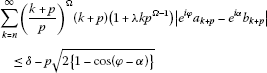(2.1)

for some $-\pi \le \phi -\alpha \le \pi$ and $\delta >p\sqrt{2\left(1-cos\left(\phi -\alpha \right)\right)}$, then $f\in {\left(\phi ,\alpha ,\delta ,\lambda ,\mathrm{\Omega }\right)}_{p}-\mathcal{N}\left(g\right)$.

Proof By virtue of (1.2), we can write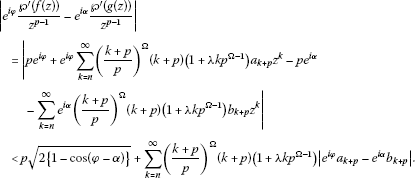If

$\sum _{k=n}^{\mathrm{\infty }}{\left(\frac{k+p}{p}\right)}^{\mathrm{\Omega }}\left(k+p\right)\left(1+\lambda k{p}^{\mathrm{\Omega }-1}\right)|{a}_{k+p}-{e}^{i\alpha }{b}_{k+p}|\le \delta -p\sqrt{2\left\{1-cos\left(\phi -\alpha \right)\right\},}$

then we see that

$|{e}^{i\phi }\frac{{\mathrm{\wp }}^{\prime }\left(f\left(z\right)\right)}{{z}^{p-1}}-{e}^{i\alpha }\frac{{\mathrm{\wp }}^{\prime }\left(g\left(z\right)\right)}{{z}^{p-1}}|<\delta \phantom{\rule{1em}{0ex}}\left(z\in U\right).$

Thus, $f\in {\left(\phi ,\alpha ,\delta ,\lambda ,\mathrm{\Omega }\right)}_{p}-\mathcal{N}\left(g\right)$. □

Example 2.2

For given

$g\left(z\right)={z}^{p}+\sum _{k=n}^{\mathrm{\infty }}{B}_{k+p}\left(\phi ,\alpha ,\delta ,\lambda ,\mathrm{\Omega }\right){z}^{k+p}\in {\mathrm{\wp }}_{\left(\mathrm{\Omega },\lambda \right)}\phantom{\rule{1em}{0ex}}\left(n,p\in \mathbb{N}=\left\{1,2,\dots \right\}\right)$

we consider

$f\left(z\right)={z}^{p}+\sum _{k=n}^{\mathrm{\infty }}{A}_{k+p}\left(\phi ,\alpha ,\delta ,\lambda ,\mathrm{\Omega }\right){z}^{k+p}\in {\mathrm{\wp }}_{\left(\mathrm{\Omega },\lambda \right)}\phantom{\rule{1em}{0ex}}\left(n,p\in \mathbb{N}=\left\{1,2,\dots \right\}\right)$

with

${A}_{k+p}=\frac{{p}^{\mathrm{\Omega }}\left(\delta -p\sqrt{2\left(1-cos\left(\phi -\alpha \right)\right)}\right)}{{\left(k+p\right)}^{\mathrm{\Omega }+2}\left(1+\lambda k{p}^{\mathrm{\Omega }-1}\right)\left(k+p-1\right)}\left(n+p-1\right){e}^{-i\phi }+{e}^{i\left(\alpha -\phi \right)}{B}_{k+p}.$

Then we have that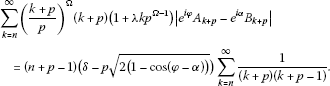(2.2)

Finally, in view of the telescopic sum, we can write

$\begin{array}{rcl}\sum _{k=n}^{\mathrm{\infty }}\frac{1}{\left(k+p\right)\left(k+p-1\right)}& =& \underset{q\to \mathrm{\infty }}{lim}\sum _{k=n}^{q}\left\{\frac{1}{\left(k+p-1\right)}-\frac{1}{\left(k+p\right)}\right\}\\ =& \underset{q\to \mathrm{\infty }}{lim}\left\{\frac{1}{\left(n+p-1\right)}-\frac{1}{\left(p+q\right)}\right\}\\ =& \frac{1}{n+p-1}.\end{array}$
(2.3)

Using (2.3) in (2.2), we have

$\sum _{k=n}^{\mathrm{\infty }}{\left(\frac{k+p}{p}\right)}^{\mathrm{\Omega }}\left(k+p\right)\left(1+\lambda k{p}^{\mathrm{\Omega }-1}\right)|{e}^{i\phi }{A}_{k+p}-{e}^{i\alpha }{B}_{k+p}|=\left(\delta -p\sqrt{2\left(1-cos\left(\phi -\alpha \right)\right)}\right).$

Therefore, $f\in {\left(\phi ,\alpha ,\delta ,\lambda ,\mathrm{\Omega }\right)}_{p}-\mathcal{N}\left(g\right)$.

Corollary 2.3 If $f\in {\mathrm{\wp }}_{\left(\mathrm{\Omega },\lambda \right)}$ satisfies

$\sum _{k=n}^{\mathrm{\infty }}{\left(\frac{k+p}{p}\right)}^{\mathrm{\Omega }}\left(k+p\right)\left(1+\lambda k{p}^{\mathrm{\Omega }-1}\right)||{a}_{k+p}|-|{b}_{k+p}||\le \delta -p\sqrt{2\left(1-cos\left(\phi -\alpha \right)\right)}$

for some $-\pi \le \phi -\alpha \le \pi$, $\delta >p\sqrt{2\left\{1-cos\left(\phi -\alpha \right)\right\}}$, and $arg\left({a}_{k+p}\right)-arg\left({b}_{k+p}\right)=\alpha -\phi$ ($n,p\in \mathbb{N}=\left\{1,2,\dots \right\}$), then $f\in {\left(\phi ,\alpha ,\delta ,\lambda ,\mathrm{\Omega }\right)}_{p}-\mathcal{N}\left(g\right)$.

Proof By Theorem 2.1, we see the inequality (2.1) which implies that $f\in {\left(\phi ,\alpha ,\delta ,\lambda ,\mathrm{\Omega }\right)}_{p}-\mathcal{N}\left(g\right)$.

Since $arg\left({a}_{k+p}\right)-arg\left({b}_{k+p}\right)=\alpha -\phi$, if $arg\left({a}_{k+p}\right)={\phi }_{k+p}$, we see $arg\left({b}_{k+p}\right)={\phi }_{k+p}-\alpha +\phi$. Therefore,

${e}^{i\phi }{a}_{k+p}-{e}^{i\alpha }{b}_{k+p}={e}^{i\phi }|{a}_{k+p}|{e}^{i{\phi }_{k+p}}-{e}^{i\alpha }|{b}_{k+p}|{e}^{i\left({\phi }_{k+p}-\alpha +\phi \right)}=\left(|{a}_{k+p}|-|{b}_{k+p}|\right){e}^{i\left({\phi }_{k+p}+\phi \right)}$

implies that

$|{e}^{i\phi }{a}_{k+p}-{e}^{i\alpha }{b}_{k+p}|=||{a}_{k+p}|-|{b}_{k+p}||.$
(2.4)

Using (2.4) in (2.1), the proof of the corollary is complete. □

Theorem 2.4 If $f\in {\mathrm{\wp }}_{\left(\mathrm{\Omega },\lambda \right)}$ satisfies

$\sum _{k=n}^{\mathrm{\infty }}{\left(\frac{k+p}{p}\right)}^{\mathrm{\Omega }}\left(1+\lambda k{p}^{\mathrm{\Omega }-1}\right)|{e}^{i\phi }{a}_{k+p}-{e}^{i\alpha }{b}_{k+p}|\le \delta -\sqrt{2\left(1-cos\left(\alpha -\phi \right)\right)}$

for some $-\pi \le \phi -\alpha \le \pi$ and $\delta >\sqrt{2\left\{1-cos\left(\phi -\alpha \right)\right\}}$, then $f\in {\left(\phi ,\alpha ,\delta ,\lambda ,\mathrm{\Omega }\right)}_{p}-\mathcal{M}\left(g\right)$.

The proof of this theorem is similar with Theorem 2.1.

Corollary 2.5 If $f\in {\mathrm{\wp }}_{\left(\mathrm{\Omega },\lambda \right)}$ satisfies

$\sum _{k=n}^{\mathrm{\infty }}{\left(\frac{k+p}{p}\right)}^{\mathrm{\Omega }}\left(1+\lambda k{p}^{\mathrm{\Omega }-1}\right)||{a}_{k+p}|-|{b}_{k+p}||\le \delta -\sqrt{2\left(1-cos\left(\phi -\alpha \right)\right)}$

for some $-\pi \le \phi -\alpha \le \pi$, $\delta >\sqrt{2\left\{1-cos\left(\phi -\alpha \right)\right\}}$, and $arg\left({a}_{k+p}\right)-arg\left({b}_{k+p}\right)=\alpha -\phi$, then $f\in {\left(\phi ,\alpha ,\delta ,\lambda ,\mathrm{\Omega }\right)}_{p}-\mathcal{M}\left(g\right)$.

Next, we derive the following theorem.

Theorem 2.6 If $f\in {\left(\phi ,\alpha ,\delta ,\lambda ,\mathrm{\Omega }\right)}_{p}-\mathcal{N}\left(g\right)$, $0\le \phi <\alpha \le \pi$ and $arg\left({e}^{i\phi }{a}_{k+p}-{e}^{i\alpha }{b}_{k+p}\right)=k\phi$, then

$\sum _{k=n}^{\mathrm{\infty }}{\left(\frac{k+p}{p}\right)}^{\mathrm{\Omega }}\left(k+p\right)\left(1+\lambda k{p}^{\mathrm{\Omega }-1}\right)|{e}^{i\phi }{a}_{k+p}-{e}^{i\alpha }{b}_{k+p}|\le \delta -p\left\{cos\phi -cos\alpha \right\}.$

Proof For $f\in {\left(\phi ,\alpha ,\delta ,\lambda ,\mathrm{\Omega }\right)}_{p}-\mathcal{N}\left(g\right)$, we have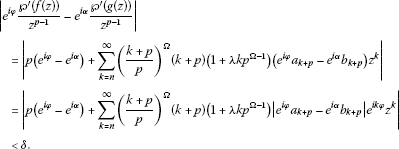Let us consider z such that $argz=-\phi$. Then ${z}^{k}=|z{|}^{k}{e}^{-ik\phi }$. For such a point $z\in U$, we see that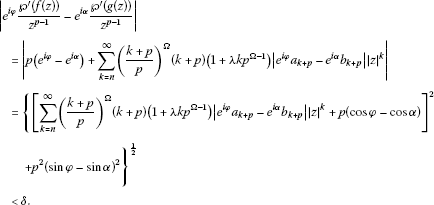This implies that

${\left\{\sum _{k=n}^{\mathrm{\infty }}{\left(\frac{k+p}{p}\right)}^{\mathrm{\Omega }}\left(k+p\right)\left(1+\lambda k{p}^{\mathrm{\Omega }-1}\right)|{e}^{i\phi }{a}_{k+p}-{e}^{i\alpha }{b}_{k+p}||z{|}^{k}+p\left(cos\phi -cos\alpha \right)\right\}}^{2}<{\delta }^{2}$

or

$p\left(cos\phi -cos\alpha \right)+\sum _{k=n}^{\mathrm{\infty }}{\left(\frac{k+p}{p}\right)}^{\mathrm{\Omega }}\left(k+p\right)\left(1+\lambda k{p}^{\mathrm{\Omega }-1}\right)|{e}^{i\phi }{a}_{k+p}-{e}^{i\alpha }{b}_{k+p}||z{|}^{k}<\delta$

for $z\in U$. Letting $|z|\to {1}^{-}$, we have that

$\sum _{k=n}^{\mathrm{\infty }}{\left(\frac{k+p}{p}\right)}^{\mathrm{\Omega }}\left(k+p\right)\left(1+\lambda k{p}^{\mathrm{\Omega }-1}\right)|{e}^{i\phi }{a}_{k+p}-{e}^{i\alpha }{b}_{k+p}|\le \delta -p\left(cos\phi -cos\alpha \right).$

□

Theorem 2.7 $f\in {\left(\phi ,\alpha ,\delta ,\lambda ,\mathrm{\Omega }\right)}_{p}-\mathcal{M}\left(g\right)$, $0\le \phi <\alpha \le \pi$ and $arg\left({e}^{i\phi }{a}_{k+p}-{e}^{i\alpha }{b}_{k+p}\right)=k\phi$, then

$\sum _{k=n}^{\mathrm{\infty }}{\left(\frac{k+p}{p}\right)}^{\mathrm{\Omega }}\left(1+\lambda k{p}^{\mathrm{\Omega }-1}\right)|{e}^{i\phi }{a}_{k+p}-{e}^{i\alpha }{b}_{k+p}|\le \delta +cos\alpha -cos\phi .$

The proof of this theorem is similar with Theorem 2.6.

Remark 2.8 Taking $\phi =0$, $\mathrm{\Omega }=0$, $\lambda =0$ and $p=1$ in Theorem 2.6, we obtain the following theorem due to Orhan et al. .

Theorem 2.9 If $f\in \left(\alpha ,\delta \right)-\mathcal{N}\left(g\right)$ and $arg\left({a}_{n}-{e}^{i\alpha }{b}_{n}\right)=\left(n-1\right)\phi$ ($n=2,3,4,\dots$), then

$\sum _{n=2}^{\mathrm{\infty }}n|{a}_{n}-{e}^{i\alpha }{b}_{n}|\le \delta +cos\alpha -1.$

Remark 2.10 Taking $\phi =0$, $\mathrm{\Omega }=0$ and $\lambda =0$ in Theorem 2.6, we obtain the following theorem due to Altuntaş et al. .

Theorem 2.11 If $f\in {\left(\alpha ,\delta \right)}_{p}-\mathcal{N}\left(g\right)$ and $arg\left({a}_{k+p}-{e}^{i\alpha }{b}_{k+p}\right)=k\phi$, then

$\sum _{k=n}^{\mathrm{\infty }}\left(k+p\right)|{a}_{k+p}-{e}^{i\alpha }{b}_{k+p}|\le \delta -p\left(1-cos\alpha \right).$

We give an application of following lemma due to Miller and Mocanu .

Lemma 2.12 Let the function

$w\left(z\right)={b}_{n}{z}^{n}+{b}_{n+1}{z}^{n+1}+{b}_{n+2}{z}^{n+2}+\cdots \phantom{\rule{1em}{0ex}}\left(n\in \mathbb{N}\right)$

be regular in the unit disk U with $w\left(z\right)\not\equiv 0$ ($z\in U$). If ${z}_{0}={r}_{0}{e}^{i{\theta }_{0}}$ (${r}_{0}<1$) and $|w\left({z}_{0}\right)|={max}_{|z|\le {r}_{0}}|w\left(z\right)|$, then ${z}_{0}{w}^{\prime }\left({z}_{0}\right)=mw\left({z}_{0}\right)$ where m is real and $m\ge n\ge 1$.

Theorem 2.13 If $f\in {\mathrm{\wp }}_{\left(\mathrm{\Omega },\lambda \right)}$ satisfies

$|{e}^{i\phi }\frac{{\mathrm{\wp }}^{\prime }\left(f\left(z\right)\right)}{{z}^{p-1}}-{e}^{i\alpha }\frac{{\mathrm{\wp }}^{\prime }\left(g\left(z\right)\right)}{{z}^{p-1}}|<\delta \left(p+n\right)-p\sqrt{2\left(1-cos\left(\phi -\alpha \right)\right)}$

for some $-\pi \le \phi -\alpha \le \pi$ and $\delta >\left(\frac{p}{p+n}\right)\sqrt{2\left(1-cos\left(\phi -\alpha \right)\right)}$, then

$|{e}^{i\phi }\frac{\mathrm{\wp }\left(f\left(z\right)\right)}{{z}^{p}}-{e}^{i\alpha }\frac{\mathrm{\wp }\left(g\left(z\right)\right)}{{z}^{p}}|<\delta +\sqrt{2\left(1-cos\left(\phi -\alpha \right)\right)}\phantom{\rule{1em}{0ex}}\left(z\in U\right).$

Proof Let us define $w\left(z\right)$ by

${e}^{i\phi }\frac{\mathrm{\wp }\left(f\left(z\right)\right)}{{z}^{p}}-{e}^{i\alpha }\frac{\mathrm{\wp }\left(g\left(z\right)\right)}{{z}^{p}}={e}^{i\phi }-{e}^{i\alpha }+\delta w\left(z\right).$
(2.5)

Then $w\left(z\right)$ is analytic in U and $w\left(0\right)=0$. By logarithmic differentiation, we obtain from (2.5) that

$\frac{{e}^{i\phi }{\mathrm{\wp }}^{\prime }\left(f\left(z\right)\right)-{e}^{i\alpha }{\mathrm{\wp }}^{\prime }\left(g\left(z\right)\right)}{{e}^{i\phi }\mathrm{\wp }\left(f\left(z\right)\right)-{e}^{i\alpha }\mathrm{\wp }\left(g\left(z\right)\right)}-\frac{p}{z}=\frac{\delta {w}^{\prime }\left(z\right)}{{e}^{i\phi }-{e}^{i\alpha }+\delta w\left(z\right)}.$

Since

$\frac{{e}^{i\phi }{\mathrm{\wp }}^{\prime }\left(f\left(z\right)\right)-{e}^{i\alpha }{\mathrm{\wp }}^{\prime }\left(g\left(z\right)\right)}{{z}^{p}\left({e}^{i\phi }-{e}^{i\alpha }+\delta w\left(z\right)\right)}=\frac{p}{z}+\frac{\delta {w}^{\prime }\left(z\right)}{{e}^{i\phi }-{e}^{i\alpha }+\delta w\left(z\right)},$

we see that

${e}^{i\phi }\frac{{\mathrm{\wp }}^{\prime }\left(f\left(z\right)\right)}{{z}^{p-1}}-{e}^{i\alpha }\frac{{\mathrm{\wp }}^{\prime }\left(g\left(z\right)\right)}{{z}^{p-1}}=p\left({e}^{i\phi }-{e}^{i\alpha }\right)+\delta w\left(z\right)\left(p+\frac{z{w}^{\prime }\left(z\right)}{w\left(z\right)}\right).$

This implies that

$|{e}^{i\phi }\frac{{\mathrm{\wp }}^{\prime }\left(f\left(z\right)\right)}{{z}^{p-1}}-{e}^{i\alpha }\frac{{\mathrm{\wp }}^{\prime }\left(g\left(z\right)\right)}{{z}^{p-1}}|=|p\left({e}^{i\phi }-{e}^{i\alpha }\right)+\delta w\left(z\right)\left(p+\frac{z{w}^{\prime }\left(z\right)}{w\left(z\right)}\right)|.$

We claim that

$|{e}^{i\phi }\frac{{\mathrm{\wp }}^{\prime }\left(f\left(z\right)\right)}{{z}^{p-1}}-{e}^{i\alpha }\frac{{\mathrm{\wp }}^{\prime }\left(g\left(z\right)\right)}{{z}^{p-1}}|<\delta \left(p+n\right)-p\sqrt{2\left(1-cos\left(\phi -\alpha \right)\right)}$

in U.

Otherwise, there exists a point ${z}_{0}\in U$ such that ${z}_{0}{w}^{\prime }\left({z}_{0}\right)=mw\left({z}_{0}\right)$ (by Miller and Mocanu’s lemma) where $w\left({z}_{0}\right)={e}^{i\theta }$ and $m\ge n\ge 1$.

Therefore, we obtain that

$\begin{array}{rcl}|{e}^{i\phi }\frac{{\mathrm{\wp }}^{\prime }\left(f\left({z}_{0}\right)\right)}{{z}_{0}^{p-1}}-{e}^{i\alpha }\frac{{\mathrm{\wp }}^{\prime }\left(g\left({z}_{0}\right)\right)}{{z}_{0}^{p-1}}|& =& |p\left({e}^{i\phi }-{e}^{i\alpha }\right)+\delta {e}^{i\theta }\left(p+m\right)|\\ \ge & \delta \left(p+m\right)-|p\left({e}^{i\phi }-{e}^{i\alpha }\right)|\\ \ge & \delta \left(p+n\right)-p\sqrt{2\left(1-cos\left(\phi -\alpha \right)\right).}\end{array}$

This contradicts our condition in Theorem 2.13. □

Hence, there is no ${z}_{0}\in U$ such that $|w\left({z}_{0}\right)|=1$. This implies that $|w\left(z\right)|<1$ for all $z\in U$. Thus, we have that

$\begin{array}{rcl}|{e}^{i\phi }\frac{\mathrm{\wp }\left(f\left(z\right)\right)}{{z}^{p}}-{e}^{i\alpha }\frac{\mathrm{\wp }\left(g\left(z\right)\right)}{{z}^{p}}|& =& |\left({e}^{i\phi }-{e}^{i\alpha }\right)+\delta w\left(z\right)|\\ \le & |{e}^{i\phi }-{e}^{i\alpha }|+\delta |w\left(z\right)|\\ <& \delta +\sqrt{2\left(1-cos\left(\phi -\alpha \right)\right)}.\end{array}$

Letting $\phi =0$, $\mathrm{\Omega }=0$, $\lambda =0$ and $\alpha =\frac{\pi }{2}$ in Theorem 2.13, we can obtain the following corollary.

Corollary 2.14 If $f\in A\left(p,n\right)$ satisfies

$|\frac{{f}^{\prime }\left(z\right)}{{z}^{p-1}}-i\frac{{g}^{\prime }\left(z\right)}{{z}^{p-1}}|<\delta \left(p+n\right)-p\sqrt{2}\phantom{\rule{1em}{0ex}}\left(z\in U\right)$

for some $\delta >\sqrt{2}\left(\frac{p}{p+n}\right)$, then

$|\frac{f\left(z\right)}{{z}^{p}}-i\frac{g\left(z\right)}{{z}^{p}}|<\delta +\sqrt{2}\phantom{\rule{1em}{0ex}}\left(z\in U\right).$

## References

1. Goodman AW: Univalent functions and nonanalytic curves. Proc. Am. Math. Soc. 1957, 8: 598–601. 10.1090/S0002-9939-1957-0086879-9

2. Ruscheweyh S: Neighborhoods of univalent functions. Proc. Am. Math. Soc. 1981, 81: 521–527. 10.1090/S0002-9939-1981-0601721-6

3. Walker JB: A note on neighborhoods of analytic functions having positive real part. Int. J. Math. Math. Sci. 1990, 13: 425–430. 10.1155/S0161171290000643

4. Owa S, Saitoh H, Nunokawa M: Neighborhoods of certain analytic functions. Appl. Math. Lett. 1993, 6: 73–77. 10.1016/0893-9659(93)90127-9

5. Altıntaş O, Owa S: Neighborhoods of certain analytic functions with negative coefficients. Int. J. Math. Math. Sci. 1996, 19: 797–800. 10.1155/S016117129600110X

6. Orhan H, Kadıoğlu E, Owa S:-Neighborhood for certain analytic functions. International Symposium on Geometric Function Theory and Applications 2007, 207–213. August 20-24

7. Altuntaş F, Owa S, Kamali M:-Neighborhood for certain class of multivalent functions. Panam. Math. J. 2009, 19(2):35–46.

8. Frasin BA:-Neighborhood for certain analytic functions with negative coefficients. Eur. J. Pure Appl. Math. 2011, 4(1):14–19.

9. Salagean G: Subclasses of univalent functions. Lecture Notes in Math. 1013. In Complex Analysis - Fifth Romanian-Finnish Seminar, Part 1 (Bucharest, 1981). Springer, Berlin; 1983:362–372.

10. Miller SS, Mocanu PT: Second order differantial inequalities in the complex plane. J. Math. Anal. Appl. 1978, 65: 289–305. 10.1016/0022-247X(78)90181-6

## Acknowledgements

Dedicated to Professor Hari M Srivastava.

## Author information

Authors

### Corresponding author

Correspondence to Fatma Sağsöz.

### Competing interests

The authors declare that they have no competing interests.

### Authors’ contributions

All authors read and approved the final manuscript.

## Rights and permissions

Reprints and Permissions

Sağsöz, F., Kamali, M. ${\left(\phi ,\alpha ,\delta ,\lambda ,\mathrm{\Omega }\right)}_{p}$-Neighborhood for some classes of multivalent functions. J Inequal Appl 2013, 152 (2013). https://doi.org/10.1186/1029-242X-2013-152